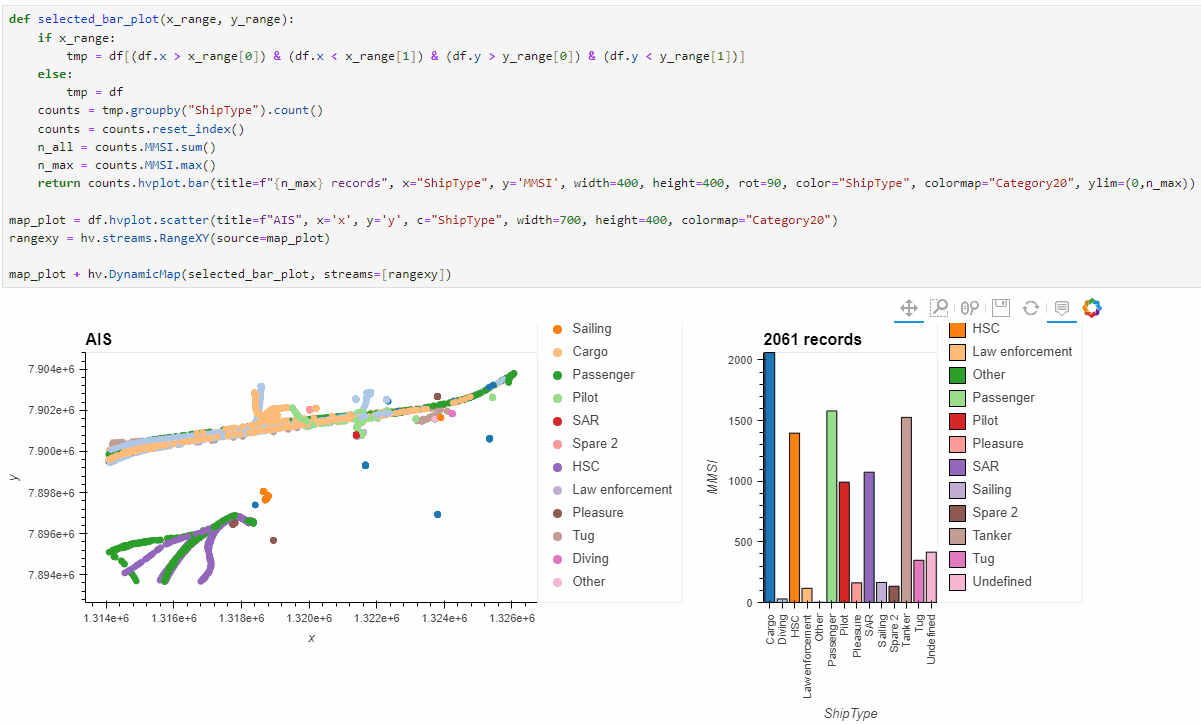# Updating axes in a DynamicMap bar chart

I am trying to link a scatterplot to a bar plot. The bar plot should update when the user zooms in the scatterplot. The basic logic is working but I cannot update the x or y axis limits. I’ve had a look at previous questions, specifically:

1. How to update limits for DynamicMap overlays? (franewise doesn’t seem to make a difference)

2. How to make DynamicMap adopt to yaxis range/limits change? - #4 by xavArtley (specific to pn.interact which I’m not using)

but I cannot figure it out.

Here’s the most relevant code snippet:

``````def selected_bar_plot(x_range, y_range):
if x_range:
tmp = df[(df.x > x_range) & (df.x < x_range) & (df.y > y_range) & (df.y < y_range)]
else:
tmp = df
counts = tmp.groupby("ShipType").count()
counts = counts.reset_index()
n_all = counts.MMSI.sum()
n_max = counts.MMSI.max()
return counts.hvplot.bar(title=f"{n_max} records", x="ShipType", y='MMSI', width=400, height=400, rot=90, color="ShipType", colormap="Category20", ylim=(0,n_max))

map_plot = df.hvplot.scatter(title=f"AIS", x='x', y='y', c="ShipType", width=700, height=400, colormap="Category20")
rangexy = hv.streams.RangeXY(source=map_plot)

map_plot + hv.DynamicMap(selected_bar_plot, streams=[rangexy])
``````